# 合并相邻序列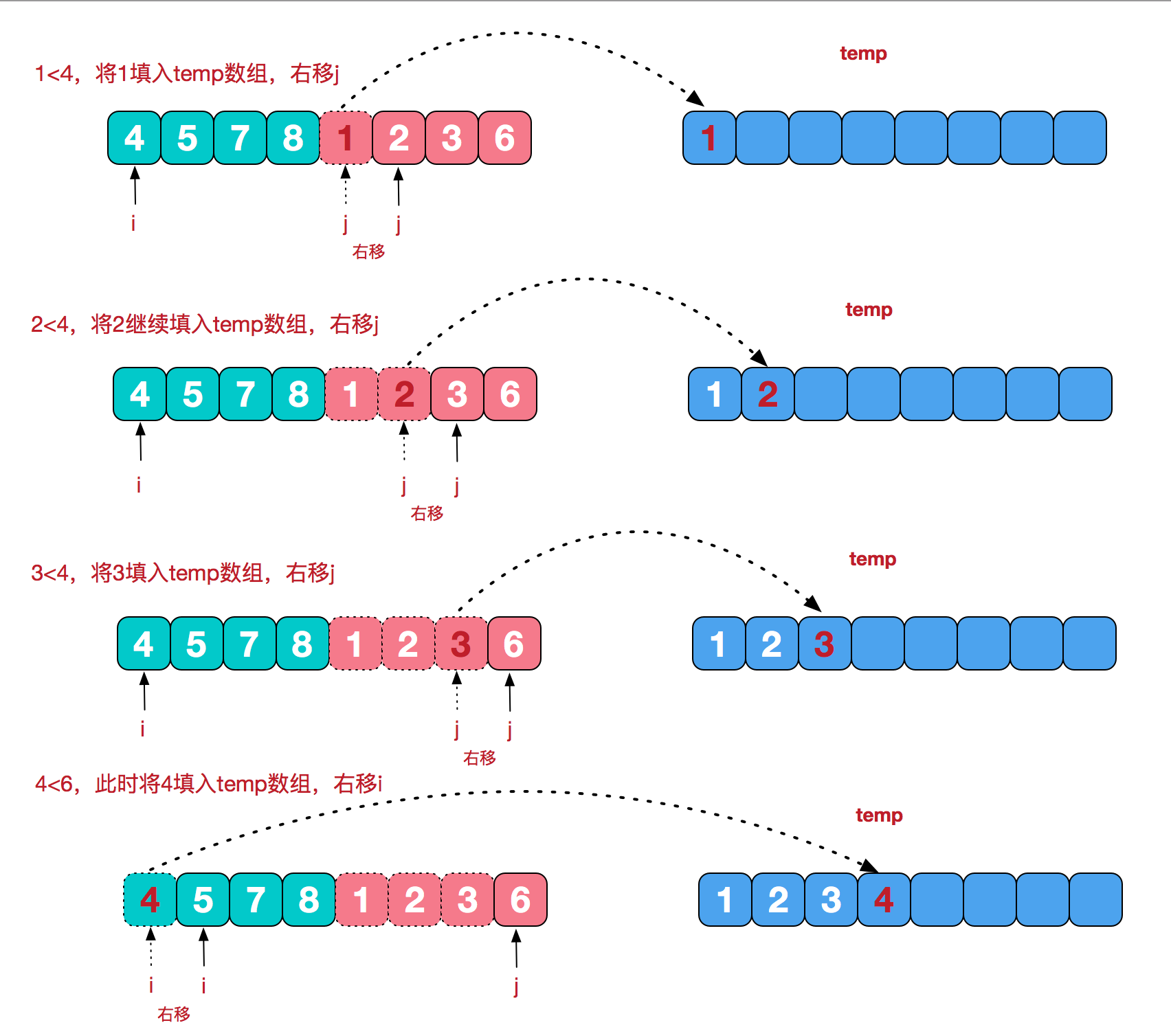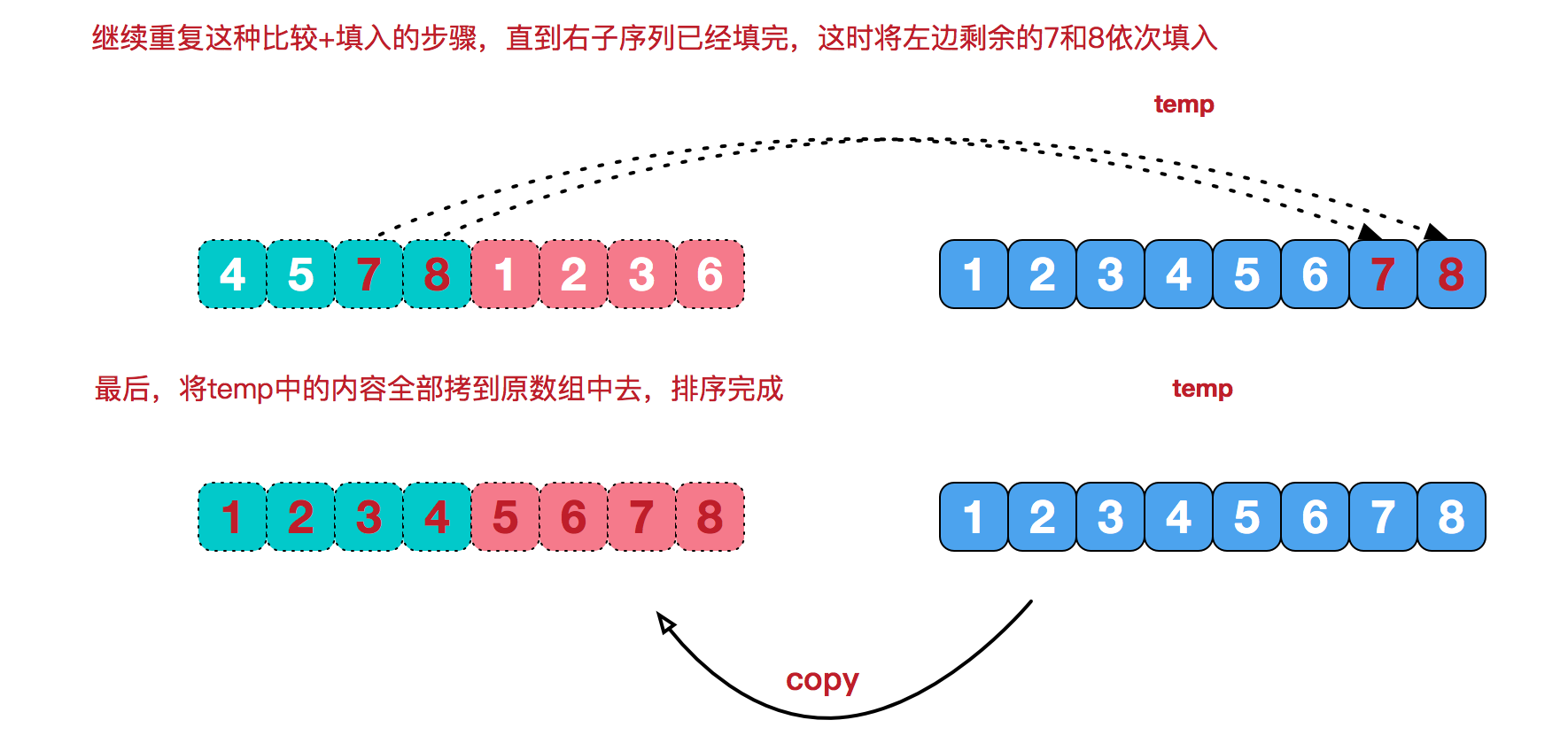# 代码实现

```package com.ikonke.openapi;

import java.util.Arrays;

public class Test2 {
public static void main(String[] args) {
int[] arr = {9, 8, 7, 6, 5, 4, 3, 2, 1};
sort(arr);
System.out.println(Arrays.toString(arr));
}

/**
* 排序递归的方法
*
* @param arr
*/
public static void sort(int[] arr) {
/**
* 临时数组
*/
int[] temp = new int[arr.length];
sort(arr, 0, arr.length - 1, temp);
}

/**
* 分治的方法
*
* @param arr
* @param left
* @param right
* @param temp
*/
private static void sort(int[] arr, int left, int right, int[] temp) {
if (left < right) {
//从数组中间开始
int mid = (left + right) / 2;
//左边归并排序，使得左子序列有序
sort(arr, left, mid, temp);

//右边归并排序，使得右子序列有序
sort(arr, mid + 1, right, temp);

//将两个有序子数组合并操作
merge(arr, left, mid, right, temp);
}
}

/**
* 数组合并
*
* @param arr   原数组
* @param left
* @param mid
* @param right
* @param temp  临时数组
*/
private static void merge(int[] arr, int left, int mid, int right, int[] temp) {
int i = left;//左指针
int j = mid + 1;//右指针
int t = 0;//临时数组指针
while (i <= mid && j <= right) {
if (arr[i] <= arr[j]) {
temp[t++] = arr[i++];
} else {
temp[t++] = arr[j++];
}
}
while (i <= mid) {//将左边数组赋值到临时数组
temp[t++] = arr[i++];
}
while (j <= right) {//将右边数组赋值到临时数组
temp[t++] = arr[j++];
}
t = 0;
//数组拷贝替换
while (left <= right) {
arr[left++] = temp[t++];
}
}
}```

[1, 2, 3, 4, 5, 6, 7, 8, 9]

# 概念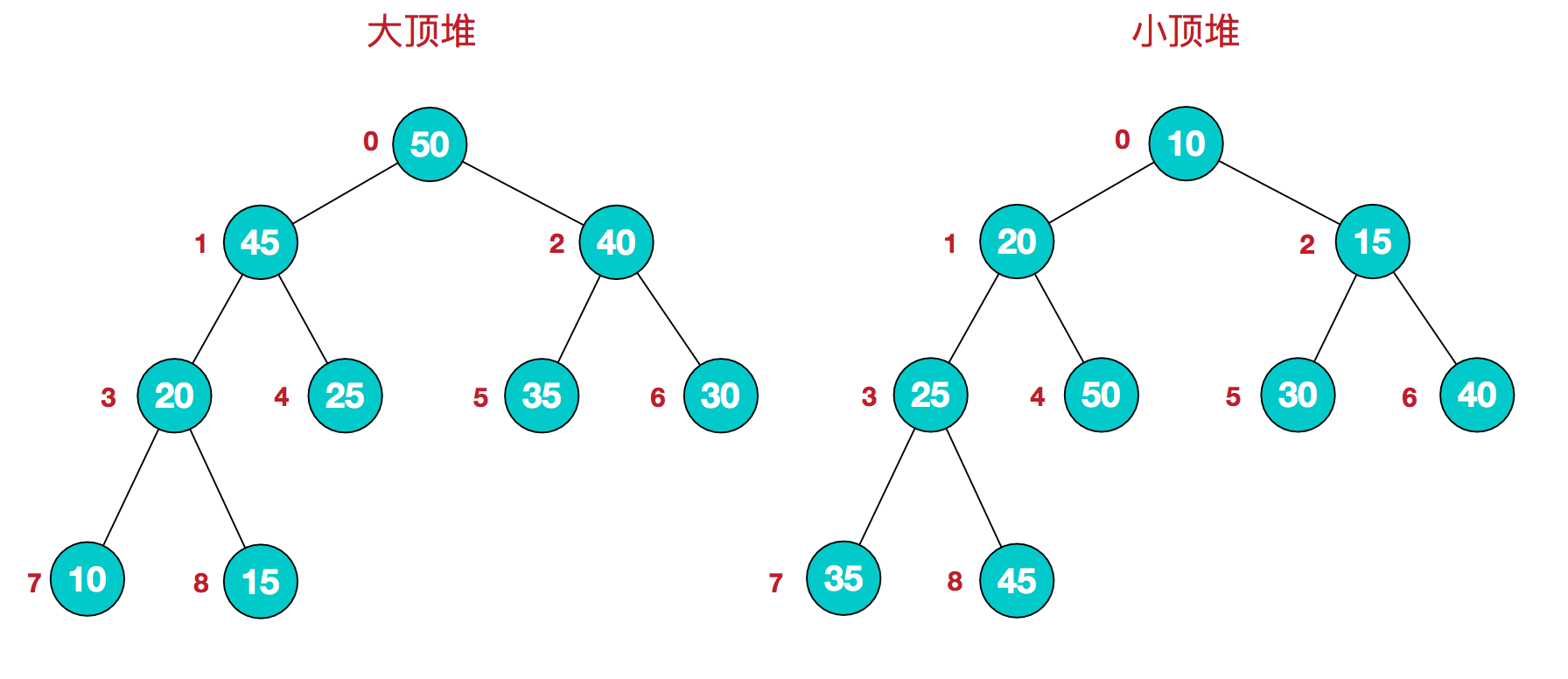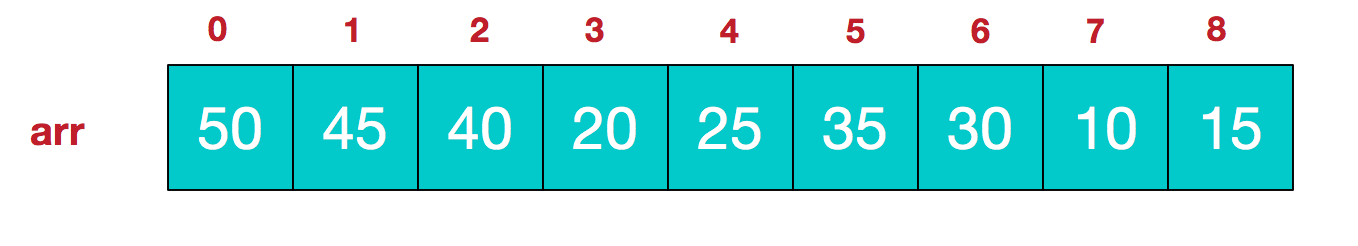# 步骤

1.构造初始堆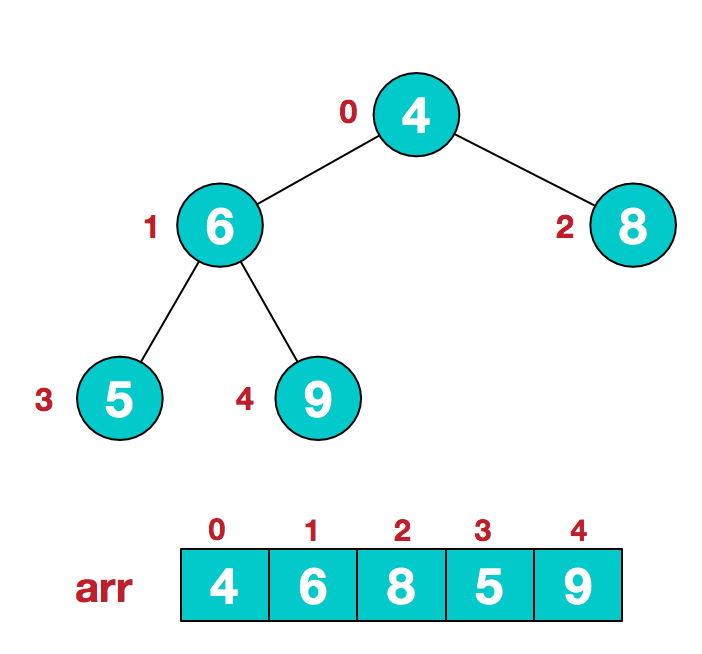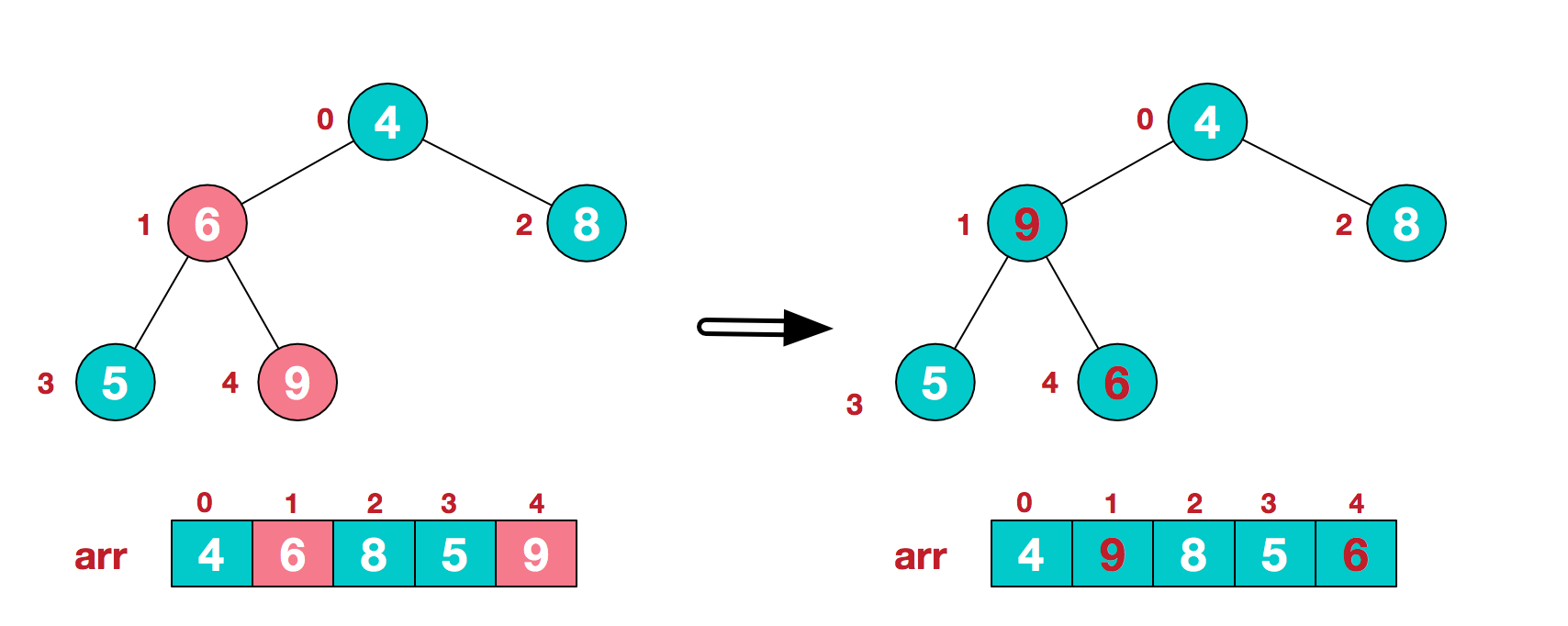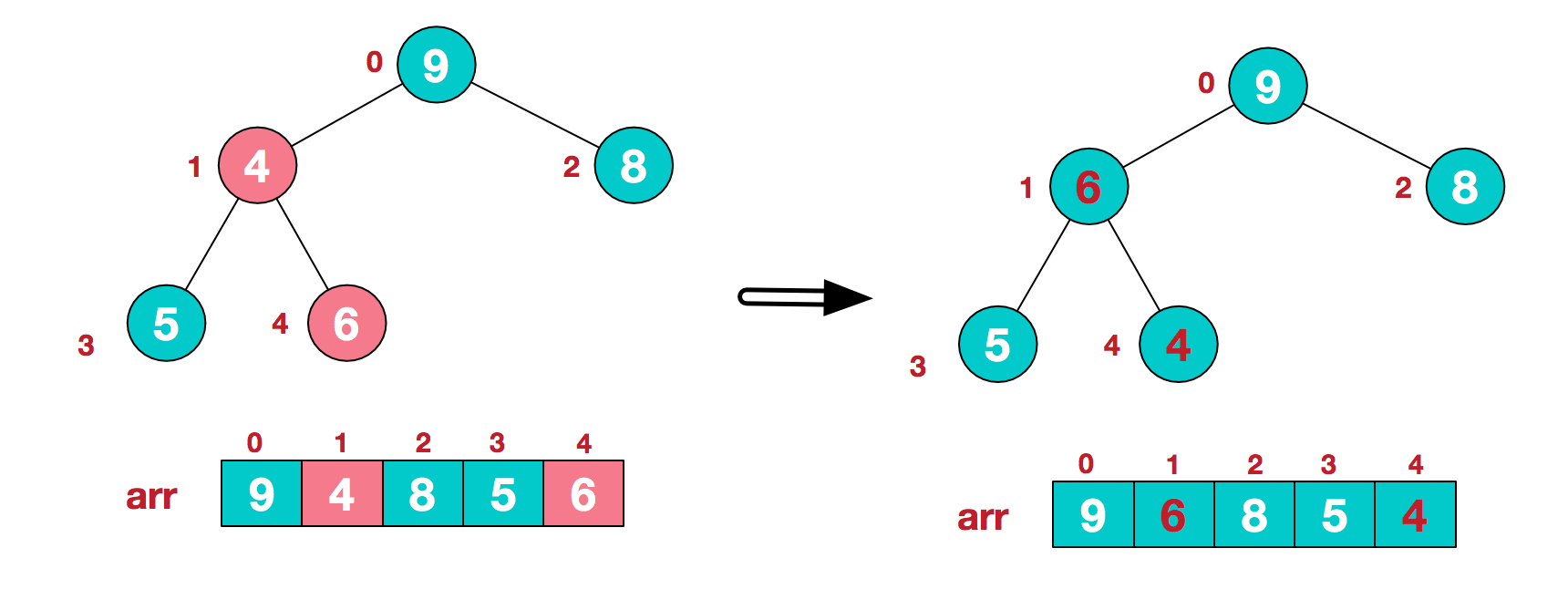2.将堆顶元素与末尾元素进行交换，使末尾元素最大。然后继续调整堆，再将堆顶元素与末尾元素交换，得到第二大元素。如此反复进行交换、重建、交换。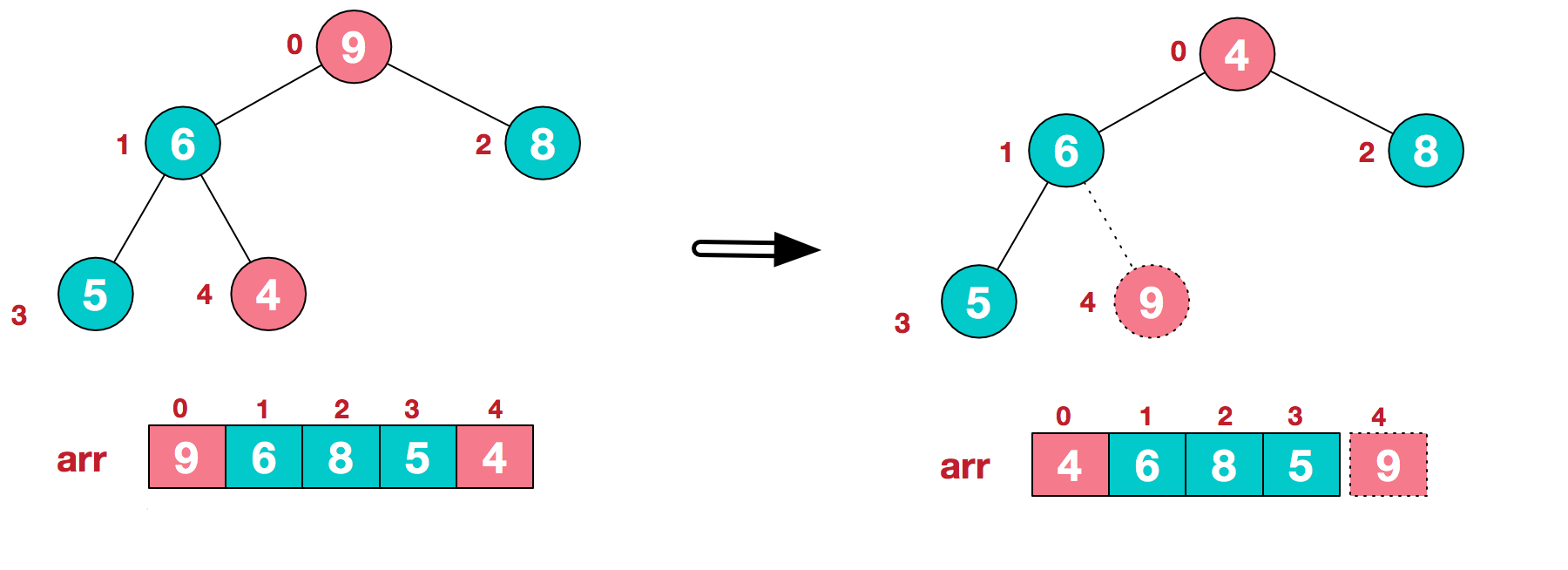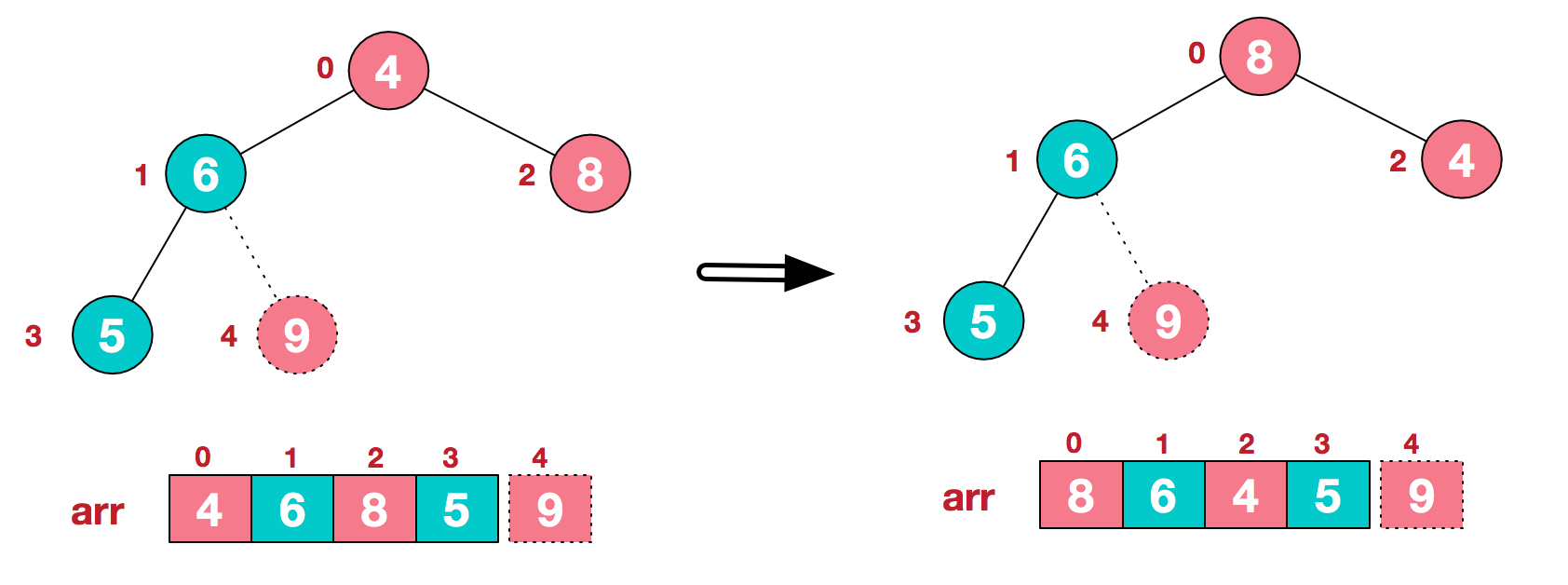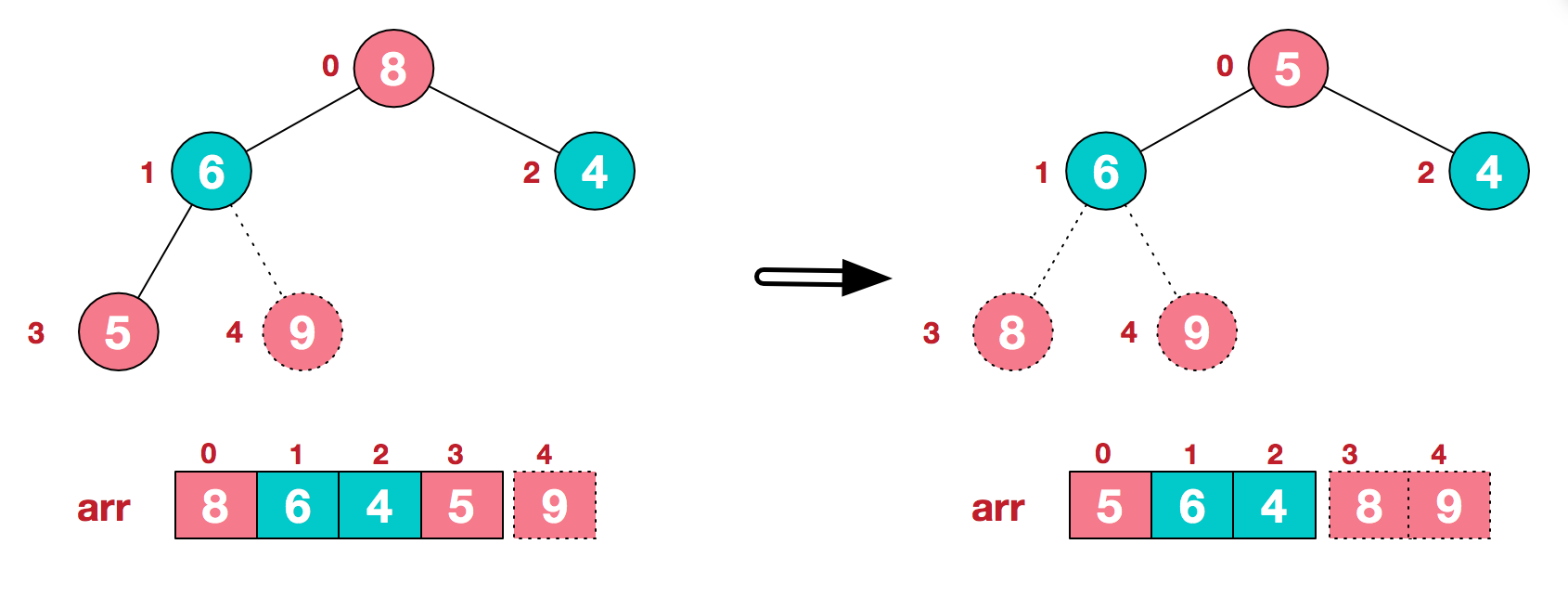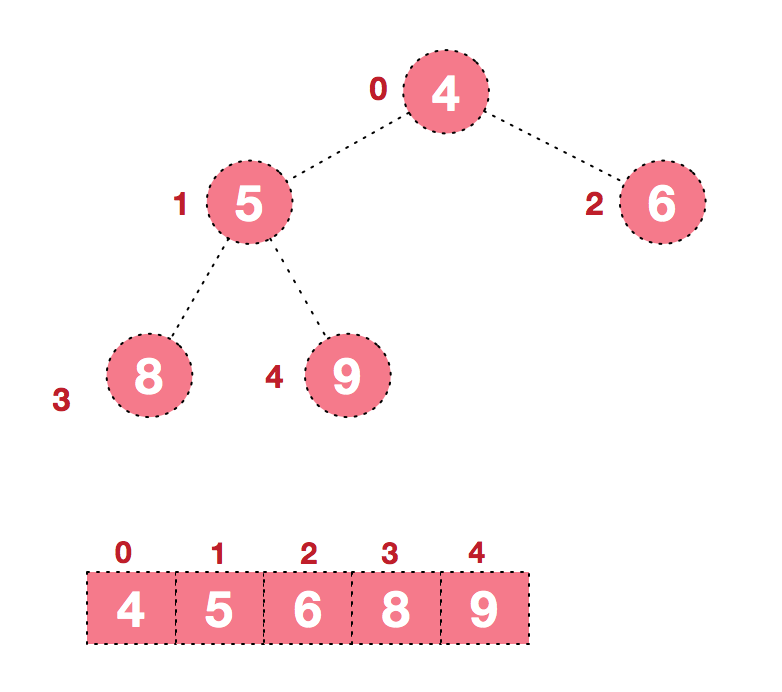## 总结堆排序的基本思路：

• 将无需序列构建成一个堆，根据升序降序需求选择大顶堆或小顶堆;
• 将堆顶元素与末尾元素交换，将最大元素”沉”到数组末端;
• 重新调整结构，使其满足堆定义，然后继续交换堆顶元素与当前末尾元素，反复执行调整+交换步骤，直到整个序列有序。

# 代码

```import java.util.Arrays;

public class Test2 {
public static void main(String[] args) {

int[] arr = {9, 8, 7, 6, 5, 4, 3, 2, 1};
sort(arr);
System.out.println(Arrays.toString(arr));
}

public static void sort(int[] arr) {
//1.构建大顶堆
for (int i = arr.length / 2 - 1; i >= 0; i--) {
//从第一个非叶子结点从下至上，从右至左调整结构
}
//2.调整堆结构+交换堆顶元素与末尾元素
for (int j = arr.length - 1; j > 0; j--) {
swap(arr, 0, j);//将堆顶元素与末尾元素进行交换
}

}

/**
* 调整大顶堆（仅是调整过程，建立在大顶堆已构建的基础上）
*
* @param arr
* @param i
* @param length
*/
public static void adjustHeap(int[] arr, int i, int length) {
int temp = arr[i];//先取出当前元素i
for (int k = i * 2 + 1; k < length; k = k * 2 + 1) {//从i结点的左子结点开始，也就是2i+1处开始
if (k + 1 < length && arr[k] < arr[k + 1]) {//如果左子结点小于右子结点，k指向右子结点
k++;
}
if (arr[k] > temp) {//如果子节点大于父节点，将子节点值赋给父节点（不用进行交换）
arr[i] = arr[k];
i = k;
} else {
break;
}
}
arr[i] = temp;//将temp值放到最终的位置
}

/**
* 交换元素
*
* @param arr
* @param a
* @param b
*/
public static void swap(int[] arr, int a, int b) {
int temp = arr[a];
arr[a] = arr[b];
arr[b] = temp;
}
}```

[1, 2, 3, 4, 5, 6, 7, 8, 9]

# 图的简介

## 图结构构成

1.顶点（vertex）：图中的数据元素，如图一。

2.边（edge）：图中连接这些顶点的线，如图一。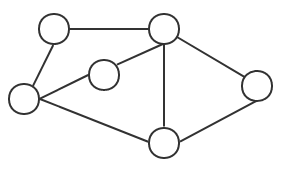G=(V,E)   或者   G=(V(G),E(G))

## 图的基本概念

### 1.无向图（undirected graph）

如果一个图结构中，所有的边都没有方向性，那么这种图便称为无向图。典型的无向图，如图二所示。由于无向图中的边没有方向性，这样我们在表示边的时候对两个顶点的顺序没有要求。例如顶点VI和顶点V5之间的边，可以表示为(V2， V6),也可以表示为(V6，V2)。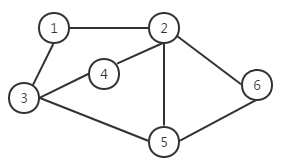V（G）= {V1，V2，V3，V4，V5，V6}

E（G）= {（V1,V2），（V1，V3），（V2，V6），（V2，V5），（V2，V4），（V4，V3），（V3，V5），（V5，V6）}

### 2.有向图（directed graph）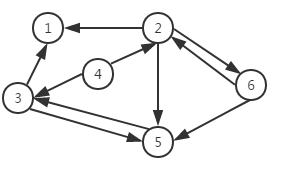V（G）= {V1，V2，V3，V4，V5，V6}

E（G）= {<V2，V1>，<V3，V1>，<V4，V3>，<V4，V2>，<V3，V5>，<V5，V3>，<V2，V5>，<V6，V5>，<V2，V6>，<V6，V2>}

*注意：无向图也可以理解成一个特殊的有向图，就是边互相指向对方节点，A指向B，B又指向A。

### 4.顶点的度

对于有向图要稍复杂些，根据连接顶点V的边的方向性，一个顶点的度有入度出度之分。

•  入度是以该顶点为端点的入边数量， 记为ID(V)。
•  出度是以该顶点为端点的出边数量， 记为OD(V)。

这样，有向图中，一个顶点V的总度便是入度和出度之和，即D(V) = ID(V) + OD(V)。例如，图三所示的有向图中，顶点V5的入度为3,出度为1，因此，顶点V5的总度为4。

### 5.邻接顶点

• 入边邻接顶点：连接该顶点的边中的起始顶点。例如，对于组成<V2，V6>这条边的两个顶点，V2是V6的入边邻接顶点。
• 出边邻接顶点：连接该顶点的边中的结束顶点。例如，对于组成<V2，V6>这条边的两个顶点，V6是V2的出边邻接顶点。

### 6.无向完全图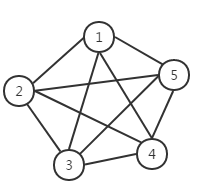### 7.有向完全图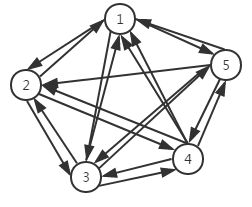### 9.无权图和有权图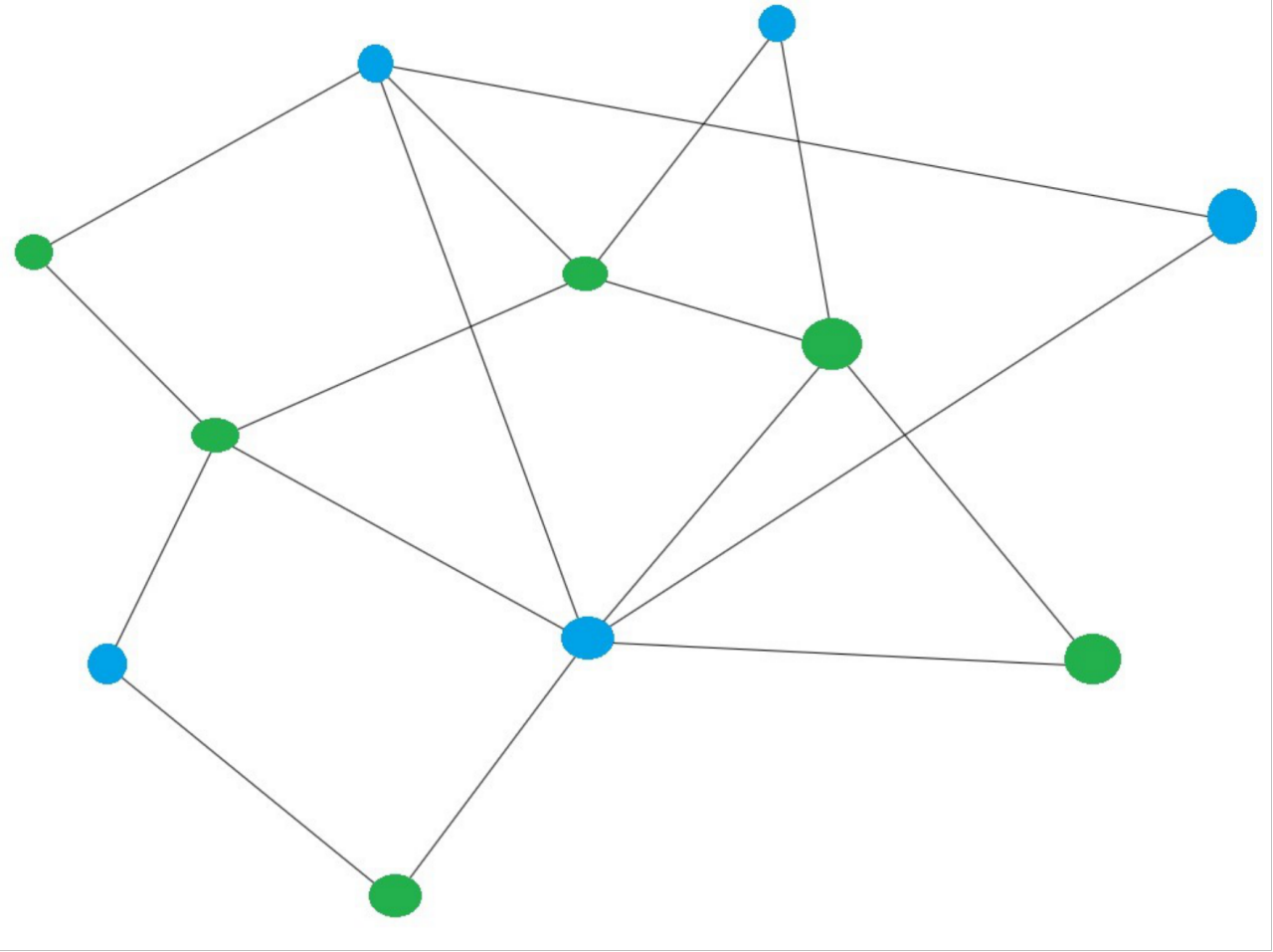### 10.图的连通性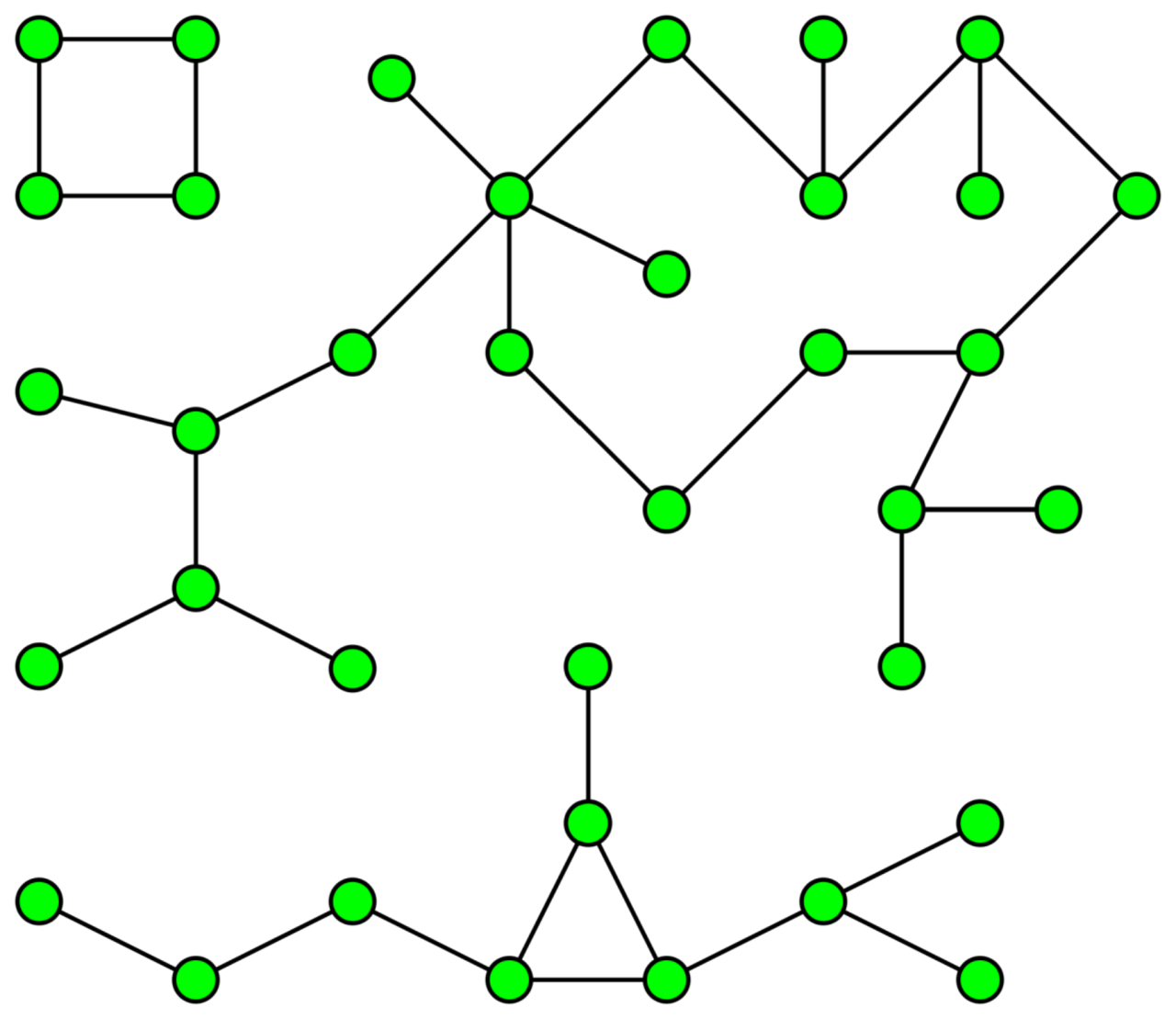### 11.简单图 ( Simple Graph)

1.自环边（self-loop）：节点自身的边，自己指向自己。

2.平行边（parallel-edges）：两个节点之间存在多个边相连接。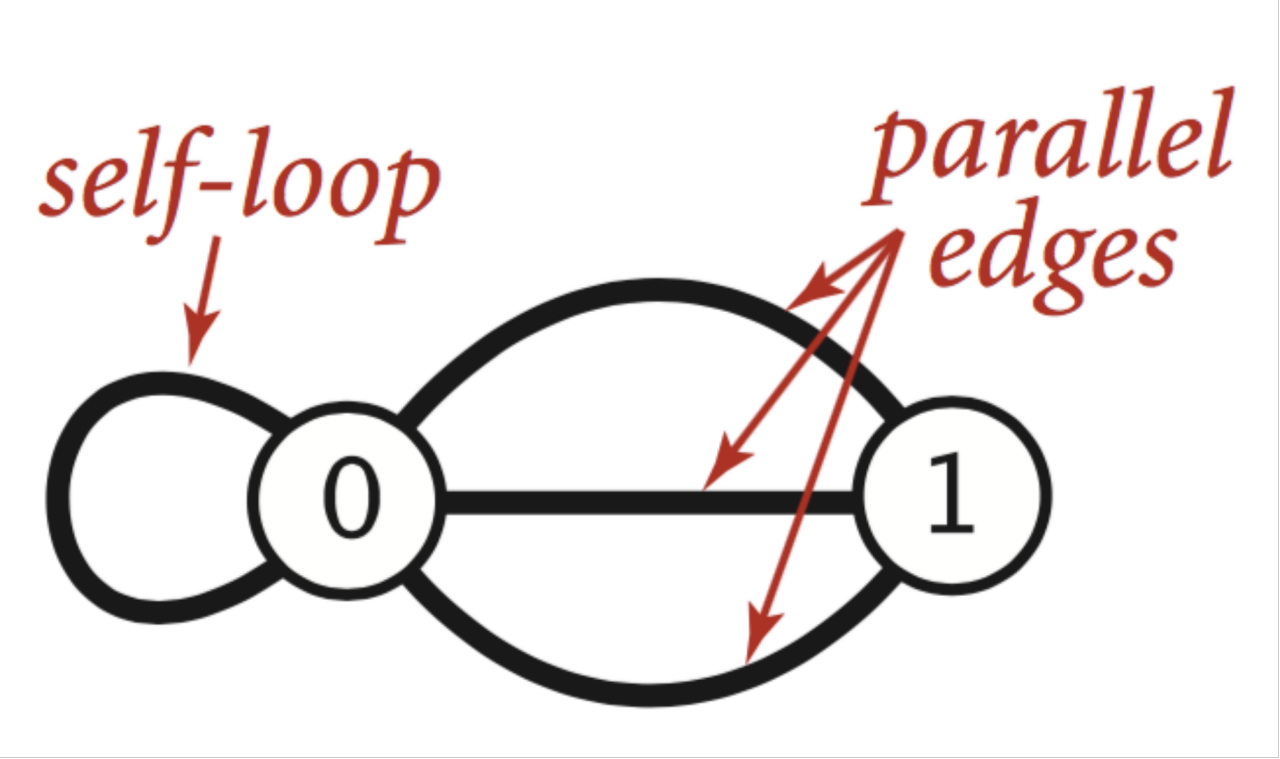## TypeScript中用for in遍历对象的属性

ts遍历对象报错误：

TS7053: Element implicitly has an ‘any’ type because expression of type ‘string’ can’t be used to index type ‘{ a: number; b: number; }’.   No index signature with a parameter of type ‘string’ was found on type ‘{ a: number; b: number; }’.

```let arr = {
a: 1,
b: 2
};

for (const n in arr) {
console.log(n, arr[n]);
}```

```{
"extends": "./tsconfig.json",
"compilerOptions": {
"suppressImplicitAnyIndexErrors": true,
"outDir": "./out-tsc/app",
"types": []
},
"files": [
"src/main.ts",
"src/polyfills.ts"
],
"include": [
"src/**/*.d.ts"
]
}```